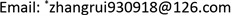﻿ i阶Lp对偶仿射表面积 The i th Lp Dual Affine Surface Area

Pure Mathematics
Vol.08 No.01(2018), Article ID:23646,6 pages
10.12677/PM.2018.81012

The i th Lp Dual Affine Surface Area

Rui Zhang1*, Tongyi Ma2

1College of Mathematics and Statistics, Northwest Normal University, Lanzhou Gansu

2College of Mathematics and Statistics, Hexi University, Zhangye GansuReceived: Jan. 8th, 2018; accepted: Jan. 23rd, 2018; published: Jan. 31st, 2018ABSTRACT

According to the Lp mixed volume, Ludwig extended the notion of Lp-affine surface area. Recently, Wang and He extended the Lp dual affine surface area. More recently, Ma studied the i-th Lp affine surface area. In this paper, we introduce the concept of i-th Lp dual affine surface area and established some inequalities according to the Brunn-Minowski-Fiery.

Keywords:Lp Affine Surface Area, Lp Dual Affine Surface Area, i-th Lp Affine Surface area, Brunn-Minkowski-Firey Theory, i-th Lp Dual Affine Surface Area

i阶Lp对偶仿射表面积

1西北师范大学数学与统计学院，甘肃 兰州

2河西学院数学与统计学院，甘肃 张掖Ludwig根据Lp混合体积的定义引进了Lp仿射表面积的概念，随后汪和何定义了Lp仿射对偶仿射表面积。近年，马统一引进了i阶Lp仿射表面积，本文介绍了的i阶Lp对偶仿射表面积的定义并且利用Brunn-Minowski-Fiery理论建立了几个不等式。1. 引言和主要结果

Leichtweiß (  )给出仿射表面积的定义，若 $K\in {\mathcal{K}}^{n}$$K$ 的仿射表面积 $\Omega \left(K\right)$ 被定义为：

${n}^{-\frac{1}{n}}{\Omega }_{p}{\left(K\right)}^{\frac{n+1}{n}}=\mathrm{inf}\left\{n{V}_{1}\left(K,{Q}^{\ast }\right)V{\left(Q\right)}^{\frac{1}{n}}:Q\in {S}_{o}^{n}\right\}$ (1.1)

Lutwak根据 ${L}_{p}$ 混合体积引进了 ${L}_{p}$ 仿射表面积(  )。若 $K\in {\mathcal{K}}_{o}^{n}$$p\ge 1$$K$${L}_{p}$ 仿射表面积 $\Omega \left(K\right)$ 被定义为：

${n}^{-\frac{p}{n}}{\Omega }_{p}{\left(K\right)}^{\frac{n+p}{n}}=\mathrm{inf}\left\{n{V}_{p}\left(K,{Q}^{\ast }\right)V{\left(Q\right)}^{\frac{p}{n}}:Q\in {S}_{o}^{n}\right\}$ (1.2)

2008年，根据 ${L}_{p}$ 混合体积的定义(  )，汪和何给出对偶仿射表面积的定义，若 $K\in {\mathcal{K}}_{o}^{n}$$1\le p\le n$${L}_{p}$ 对偶仿射表面积 ${\stackrel{˜}{\Omega }}_{-p}\left(K\right)$ 被定义为：

${n}^{\frac{p}{n}}{\stackrel{˜}{\Omega }}_{-p}{\left(K\right)}^{\frac{n-p}{n}}=\mathrm{inf}\left\{n{\stackrel{˜}{V}}_{-p}\left(K,{Q}^{\ast }\right)V{\left(Q\right)}^{-\frac{p}{n}}:Q\in {S}_{o}^{n}\right\}$ (1.3)

$K\in {\mathcal{K}}_{o}^{n}$$i\in \left\{0,1,\cdots ,n\right\}$$i$${L}_{p}$ 仿射表面积 ${\Omega }_{p}^{i}\left(K\right)$ 被定义为：

${n}^{-\frac{p}{n-i}}{\Omega }_{p}^{\left(i\right)}{\left(K\right)}^{\frac{n+p-i}{n-i}}=\mathrm{inf}\left\{n{W}_{p,i}\left(K,{Q}^{\ast }\right){\stackrel{˜}{W}}_{i}{\left(Q\right)}^{\frac{p}{n-i}}:Q\in {S}_{o}^{n}\right\}$ (1.4)

${\stackrel{˜}{\Omega }}_{p}^{\left(i\right)}\left(K\right)={\int }_{{S}_{n-1}}{f}_{p,i}{\left(K,u\right)}^{\frac{n-i}{n+p-i}}\text{d}S\left(u\right)$ (1.5)

${n}^{\frac{p}{n-i}}{\stackrel{˜}{\Omega }}_{-p}^{\left(i\right)}{\left(K\right)}^{\frac{n-p-i}{n-i}}=\mathrm{inf}\left\{n{\stackrel{˜}{W}}_{-p,i}\left(K,{Q}^{\ast }\right){\stackrel{˜}{W}}_{i}{\left(Q\right)}^{-\frac{p}{n-i}}:Q\in {S}_{o}^{n}\right\}$ (1.6)

${\stackrel{˜}{\Omega }}_{-p}^{\left(i\right)}{\left(K\right)}^{n-p-i}\le {n}^{n-p-i}{\omega }_{n}^{-2p}{W}_{i}{\left(K\right)}^{n-i}{\stackrel{˜}{W}}_{i}{\left(K\right)}^{p}$ (1.7)

$i=0$ 时，等号成立当且仅当 $K$ 是一个椭球体，当 $0 时，当且仅当 $K$ 是一个中心在原点的 $n$ 维球。

${\stackrel{˜}{\Omega }}_{-p}^{\left(i\right)}{\left(K\right)}^{n-p-i}\le {n}^{n-p-i}{\left({\omega }_{i}{\omega }_{n-i}\right)}^{-2p}{\left(\begin{array}{c}n\\ i\end{array}\right)}^{2p}{W}_{i}{\left(K\right)}^{n+p-i}$ (1.8)

$i=0$ 时，等号成立当且仅当 $K$ 是一个椭球体，当 $0 时，当且仅当 $K$ 是一个中心在原点的 $\left(n-i\right)$ 维球。

${\stackrel{˜}{\Omega }}_{-p}^{\left(i\right)}\left(K\right){\stackrel{˜}{\Omega }}_{-p}^{\left(i\right)}\left({K}^{\ast }\right)\le {n}^{2}{W}_{i}\left(K\right){\stackrel{˜}{W}}_{i}\left({K}^{\ast }\right)$ (1.9)

$i=0$ 时，等号成立当且仅当 $K$ 是一个中心在原点的椭球体，当 $0 时，当且仅当 $K$ 是一个中心在原点的球。

2. 预备知识

$h\left(K,x\right)=\mathrm{max}\left\{x\cdot y:y\in K\right\},\text{\hspace{0.17em}}x\in {ℝ}^{n}$ (2.1)

$\rho \left(K,x\right)=\mathrm{max}\left\{\lambda >0:\lambda x\in K\right\},x\in {ℝ}^{n}\\left\{0\right\}$ (2.2)

${K}^{\ast }=\left\{x\in {ℝ}^{n}:x\cdot y\le 1,y\in K\right\}$ (2.3)

${h}_{{K}^{\ast }}=\frac{1}{{\rho }_{K}}$${\rho }_{{K}^{\ast }}=\frac{1}{{h}_{K}}$ (2.4)

$V\left(K\right)V\left({K}^{\ast }\right)\le {\omega }_{n}^{2}$ (2.5)

$V\left(K\right)=\frac{1}{n}{\int }_{{S}^{n-1}}{\rho }_{K}^{n}\left(u\right)\text{d}S\left(u\right)$ (2.6)

$\rho {\left(\lambda \cdot K+p\mu \cdot L,\cdot \right)}^{p}=\lambda \rho {\left(K,\cdot \right)}^{p}=\mu \rho {\left(L,\cdot \right)}^{p}$ (2.7)

1996年，给出了 ${L}_{p}$ 对偶混合体积的概念(  )：如果 $K,L\in {S}_{o}^{n}$$p\ge 1$$\epsilon >0$$K$$L$${L}_{p}$ 对偶混合体积被定义为：

$\frac{n}{-p}{\stackrel{˜}{V}}_{-p}\left(K,L\right)=\underset{\epsilon \to 0}{\mathrm{lim}}\frac{V\left(K+{}_{-p}\epsilon \cdot L\right)-V\left(K\right)}{\epsilon }$ (2.8)

${\stackrel{˜}{V}}_{-p}\left(K,L\right)=\frac{1}{n}{\int }_{{S}^{n-1}}{\rho }_{K}^{n+p}\left(u\right){\rho }_{L}^{-p}\left(u\right)\text{d}S\left(u\right)$ (2.9)

${\stackrel{˜}{V}}_{-p}\left(K,K\right)=V\left(K\right)$ (2.10)

${L}_{p}$ 对偶混合均质积分的定义如下：如果 $K,L\in {S}_{o}^{n}$$p\ge 1$$\epsilon >0$ 且实数 $i\ne n$$K$$L$${L}_{p}$ 对偶混合均质积分 ${\stackrel{˜}{W}}_{-p,i}\left(K,L\right)$ 被定义为(  )：

$\frac{n-i}{-p}{\stackrel{˜}{W}}_{-p,i}\left(K,L\right)=\underset{\epsilon \to {0}^{+}}{\mathrm{lim}}\frac{{\stackrel{˜}{W}}_{i}\left(K+{}_{-p}\epsilon \cdot L\right)-{\stackrel{˜}{W}}_{i}\left(K\right)}{\epsilon }$ (2.11)

$i=0$ ，则(2.11)就是经典的 ${L}_{p}$ 对偶混合体积，即 ${\stackrel{˜}{W}}_{-p,0}\left(K,L\right)={\stackrel{˜}{V}}_{-p}\left(K,L\right)$

${\stackrel{˜}{W}}_{-p,i}\left(K,L\right)=\frac{1}{n}{\int }_{{S}^{n-1}}{\rho }_{K}^{n+p-i}\left(u\right){\rho }_{L}^{-p}\left(u\right)\text{d}S\left(u\right)$ (2.12)

${\stackrel{˜}{W}}_{-p,i}\left(K,K\right)={\stackrel{˜}{W}}_{i}\left(K\right)$ (2.13)

${\stackrel{˜}{W}}_{i}\left(K\right)\le V{\left(K\right)}^{\frac{n-i}{n}}{\omega }_{n}^{\frac{i}{n}}$ (2.14)

${\stackrel{˜}{W}}_{i}\left(K\right)\le {W}_{i}\left(K\right)$ (2.15)

3. 定理1.1~1.3的证明

${\stackrel{˜}{\Omega }}_{-p}^{\left(i\right)}{\left(K\right)}^{n-p-i}\le {n}^{n-p-i}{\stackrel{˜}{W}}_{-p,i}{\left(K,{Q}^{\ast }\right)}^{n-i}{\stackrel{˜}{W}}_{i}{\left(Q\right)}^{-p}$

$Q={K}^{\ast }$ ，则有

${\stackrel{˜}{\Omega }}_{-p}^{\left(i\right)}{\left(K\right)}^{n-p-i}\le {n}^{n-p-i}{\stackrel{˜}{W}}_{i}{\left(K\right)}^{n-i}{\stackrel{˜}{W}}_{i}{\left({K}^{\ast }\right)}^{-p}$ (3.1)

$\begin{array}{c}{\stackrel{˜}{\Omega }}_{-p}^{\left(i\right)}{\left(K\right)}^{n-p-i}{\stackrel{˜}{W}}_{i}{\left(K\right)}^{-p}\le {n}^{n-p-i}{\stackrel{˜}{W}}_{i}{\left(K\right)}^{n-i}\left({\stackrel{˜}{W}}_{i}{\left({K}^{\ast }\right)}^{-p}{\stackrel{˜}{W}}_{i}{\left(K\right)}^{-p}\right)\\ \le {n}^{n-p-i}{\stackrel{˜}{W}}_{i}{\left(K\right)}^{n-i}{\omega }_{n}^{\frac{-2ip}{n}}\left(V{\left(K\right)}^{-p}V{\left({K}^{\ast }\right)}^{\frac{-\left(n-ip}{n}}\right)\\ \le {n}^{n-p-i}{\omega }_{n}^{-2p}{\stackrel{˜}{W}}_{i}{\left(K\right)}^{n-i}\end{array}$

${\stackrel{˜}{\Omega }}_{-p}^{\left(i\right)}{\left(K\right)}^{n-p-i}\le {n}^{n-p-i}{\omega }_{n}^{-2p}{\stackrel{˜}{W}}_{i}{\left(K\right)}^{n-i}{\stackrel{˜}{W}}_{i}{\left(K\right)}^{p}$

$\begin{array}{c}{\stackrel{˜}{\Omega }}_{-p}^{\left(i\right)}{\left(K\right)}^{n-p-i}\le {n}^{n-p-i}{\stackrel{˜}{W}}_{i}{\left(K\right)}^{n-i}\stackrel{˜}{{W}_{i}}{\left(}^{{K}^{\ast }}\\ ={n}^{n-p-i}{\stackrel{˜}{W}}_{i}{\left(K\right)}^{n+p-i}{\left[{\stackrel{˜}{W}}_{i}\left(K\right){\stackrel{˜}{W}}_{i}\left({K}^{\ast }\right)\right]}^{-p}\\ ={n}^{n-p-i}{\stackrel{˜}{W}}_{i}{\left(K\right)}^{n+p-i}{\omega }_{i}^{-2p}{\left(\begin{array}{c}n\\ i\end{array}\right)}^{2p}{\left[{V}_{n-i}\left(K\right){V}_{n-i}\left({K}^{\ast }\right)\right]}^{-p}\\ \le {n}^{n-p-i}{\left({\omega }_{i}{\omega }_{n-i}\right)}^{-2p}{\left(\begin{array}{c}n\\ i\end{array}\right)}^{2p}{\omega }_{n}{}^{-2p}{\stackrel{˜}{W}}_{i}{\left(K\right)}^{n+p-i}\end{array}$

${n}^{\frac{p}{n-i}}{\stackrel{˜}{\Omega }}_{-p}^{\left(i\right)}{\left(K\right)}^{\frac{n-p-i}{n-i}}\le n{\stackrel{˜}{W}}_{-p,i}\left(K,{Q}^{\ast }\right){\stackrel{˜}{W}}_{i}{\left(Q\right)}^{\frac{-p}{n-i}}$

${n}^{\frac{p}{n-i}}{\stackrel{˜}{\Omega }}_{-p}^{\left(i\right)}{\left(K\right)}^{\frac{n-p-i}{n-i}}\le n{\stackrel{˜}{W}}_{i}\left(K\right){\stackrel{˜}{W}}_{i}{\left({K}^{\ast }\right)}^{\frac{-p}{n-i}}$ (3.2)

$K={K}^{\ast }$ ，我们有

${n}^{\frac{p}{n-i}}{\stackrel{˜}{\Omega }}_{-p}^{\left(i\right)}{\left({K}^{\ast }\right)}^{\frac{n-p-i}{n-i}}\le n{\stackrel{˜}{W}}_{i}\left({K}^{\ast }\right){\stackrel{˜}{W}}_{i}{\left(K\right)}^{\frac{-p}{n-i}}$ (3.3)

$\begin{array}{l}{\left({\stackrel{˜}{\Omega }}_{-p}^{\left(i\right)}\left(K\right){\stackrel{˜}{\Omega }}_{-p}^{\left(i\right)}\left({K}^{\ast }\right)\right)}^{\frac{n-p-i}{n-i}}\\ \le {n}^{\frac{2\left(n-p-i\right)}{n-i}}{\stackrel{˜}{W}}_{i}\left(K\right){\stackrel{˜}{W}}_{i}\left({K}^{\ast }\right){\left({\stackrel{˜}{W}}_{i}\left(K\right){\stackrel{˜}{W}}_{i}\left({K}^{\ast }\right)\right)}^{{}^{\frac{-p}{n-i}}}\\ \le {n}^{\frac{2\left(n-p-i\right)}{n-i}}{\left({\stackrel{˜}{W}}_{i}\left(K\right){\stackrel{˜}{W}}_{i}\left({K}^{\ast }\right)\right)}^{{}^{\frac{n-p-i}{n-i}}}\end{array}$

${\stackrel{˜}{\Omega }}_{-p}^{\left(i\right)}\left(K\right){\stackrel{˜}{\Omega }}_{-p}^{\left(i\right)}\left({K}^{\ast }\right)\le {n}^{2}{\stackrel{˜}{W}}_{i}\left(K\right){\stackrel{˜}{W}}_{i}\left({K}^{\ast }\right)$

The i th Lp Dual Affine Surface Area[J]. 理论数学, 2018, 08(01): 99-104. http://dx.doi.org/10.12677/PM.2018.81012

1. 1. Leichtweiß, K. (1989) Bemerkungen Zur Definition Einer Erweiterten Affinoberfläche Von E. Lutwak. Manuscripta Mathematica, 65, 181-197. https://doi.org/10.1007/BF01168298

2. 2. Lutwek, E. (1996) The Brunn-Minkowsk-Firey Theoy II: Affine and Geominimal Surface Areas. Advanced Mathematics, 118, 244-294. https://doi.org/10.1006/aima.1996.0022

3. 3. Wang, W. and He, B.W. (2008) -Dual Affine Surface Area. Journal of Mathematical Analysis and Applications, 348, 746-751. https://doi.org/10.1016/j.jmaa.2008.08.006

4. 4. Ma, T.Y. (2013) Some Inequalities Related to -Type Mixed Affine Surface and Mixed Curvature Image. Journal of Inequalities and Applications, 470. https://doi.org/10.1186/1029-242X-2013-470

5. 5. Ma, T.Y. and Feng, Y.B. (2015) The th -Affine Surface Area. Journal of Inequalities and Applications, 187. https://doi.org/10.1186/s13660-015-0703-7

6. 6. Lutwek, E. (1987) Mixed Affine Surface Area. Journal of Mathematical Anal-ysis and Applications, 125, 351-360. https://doi.org/10.1016/0022-247X(87)90097-7

7. 7. Gardner, R.J. (2006) Geometric Tomography. 2nd Edition. Cambridge University Press, Cambridge. https://doi.org/10.1017/CBO9781107341029

8. 8. Schneider, R. (1993) Convex Bodies: The Brunn-Minkowski Theory. Cam-bridge University Press, Cambridge. https://doi.org/10.1017/CBO9780511526282

9. 9. Wang, W.D. and Leng, G.S. (2005) -Dual Mixed Quermassintegrals. Indian Journal of Pure and Applied Mathematics, 36, 177-188.

10. 10. Lutwek, E. (1975) Dual Mixed Volumes. Pacific Journal of Mathematics, 58, 531-538. https://doi.org/10.2140/pjm.1975.58.531